# Plotting Functions and Graphs in Wolfram|Alpha

May 17, 2011 —Comments Off

Plotting functions in the Cartesian plane is such a simple task with Wolfram|Alpha: just enter the function you are looking to graph, and within seconds you will have a beautiful result. If you are feeling daring, enter a multivariate function, and the result will be a 3D Cartesian graph. Wolfram|Alpha is certainly not limited to Cartesian plotting; we have the functionality to make number lines, 2D and 3D polar plots, 2D and 3D parametric plots, 2D and 3D contour plots, implicit plots, log plots, log-linear plots, matrix plots, surface of revolution plots, region plots, list plots, pie charts, histograms, and more. Furthermore, in Wolfram|Alpha we can generate specialized plots for illustrating asymptotes, cusps, maxima, minima, inflection points, saddle points, solutions of ordinary differential equations, poles, eigenvalues, series expansions, definite integrals, 2D inequalities, interpolating polynomials, least-squares best fits, and more. Let’s take a look at the plotting functionality in Wolfram|Alpha, some of which is newly improved!

We will start simple with 2D Cartesian plots.

Here we plot sin(√7x)+19cos(x) for x between -20 and 20.

If we change √7 to √-7, then we get a plot of the real and imaginary parts.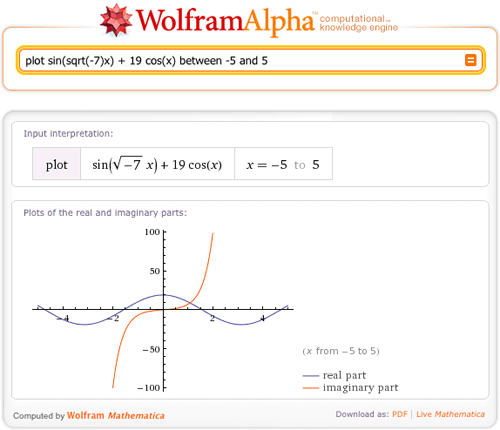In both these examples we have given Wolfram|Alpha a horizontal plot range. What happens if we don’t give Wolfram|Alpha a range?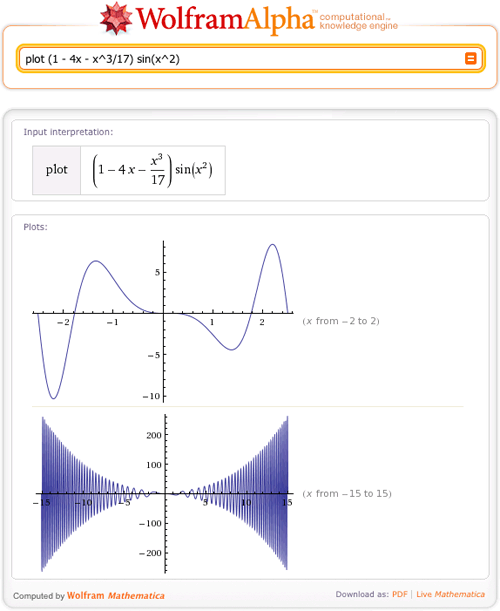We still get back a plot with all of its defining features. One of the unique features of Wolfram|Alpha is the functionality to automatically guess an appropriate plot range for univariate and bivariate functions. Here is another example: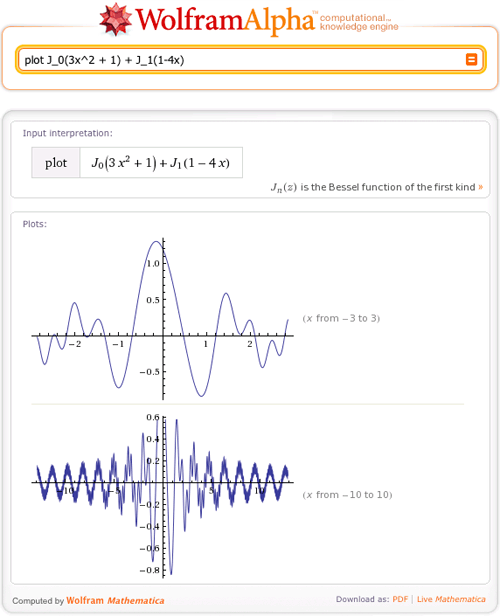So far we have told Wolfram|Alpha that we’re specifically requesting a plot. If we simply enter a univariate expression without the prefix “plot”, then we’ll always get a Cartesian plot in addition to a number of other pieces of information. Try: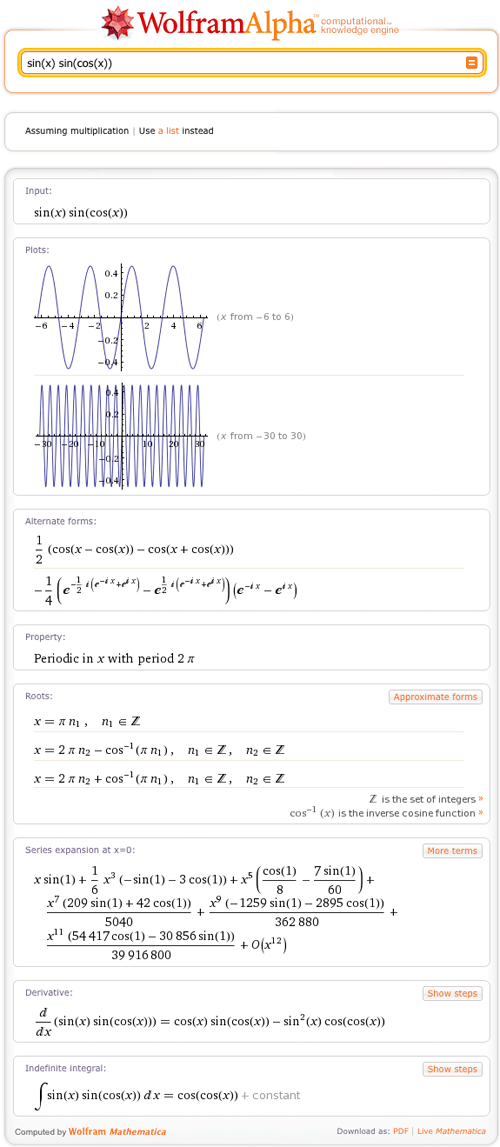Versus: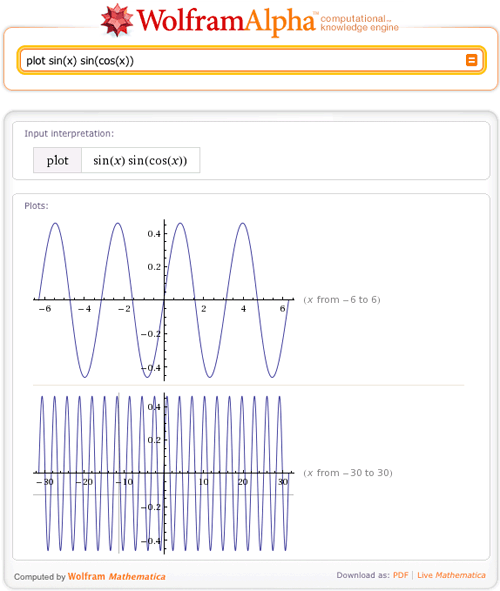One important difference is that the image sizes are larger if you specifically ask for a plot.

You can also plot more than one function at a time.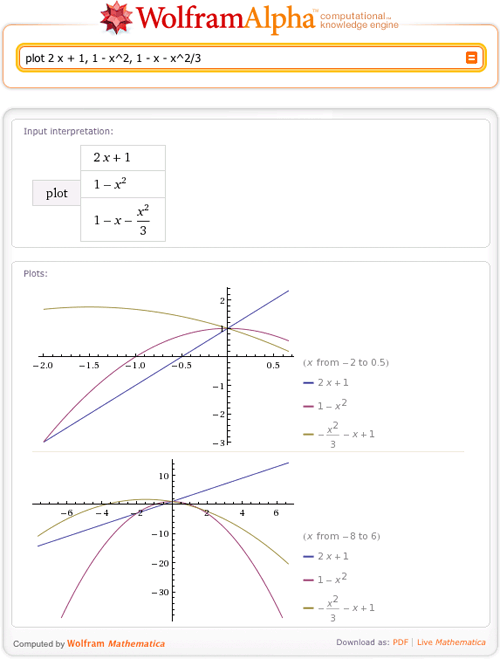The underlying Mathematica function used in all the examples is Plot. By clicking the bottom left of the images and then “Copyable plaintext”, you can see the Mathematica code used to generate the plots. For example: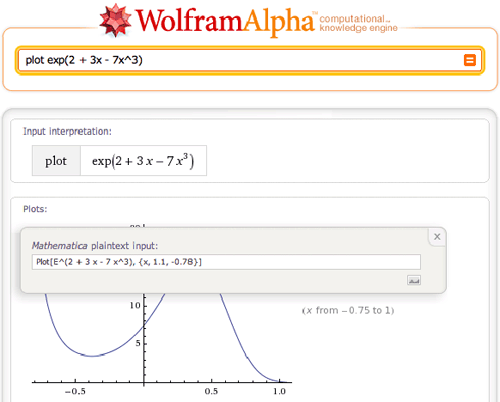The code can then be evaluated in Mathematica.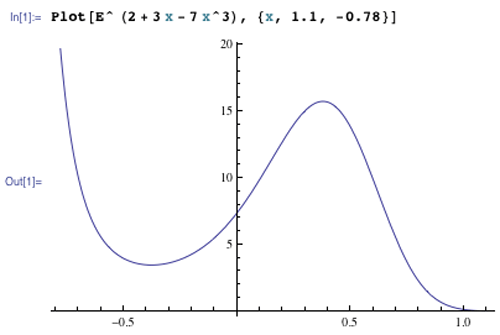Now let’s give Wolfram|Alpha a challenge and plot bivariate functions. Start by plotting y^2 cos(x) for x between -6 and 6 and y between -2 and 2.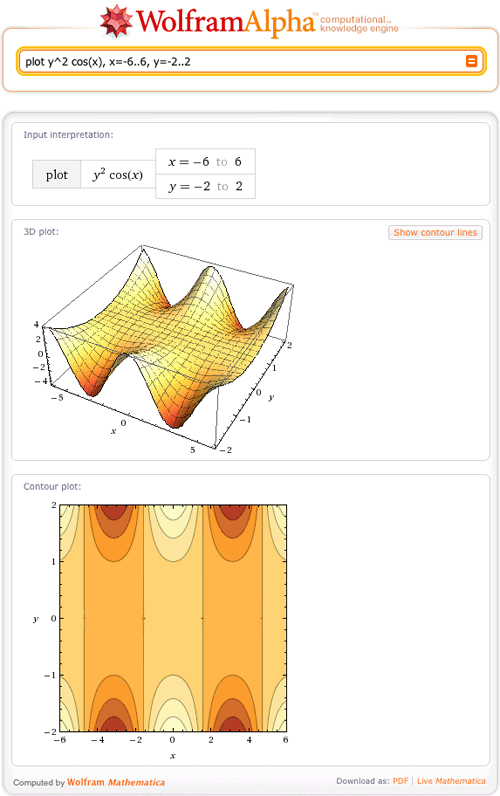As in the univariate case, Wolfram|Alpha has the capability to find an appropriate plot range for a bivariate function, the code for which is under continuous development. If Wolfram|Alpha fails to generate a plot, then it’s most likely because the code that determines a plot range has been unable to
find a region where the function has interesting behavior. In such cases you can always manually enter a plot range like we did in the example above. Here are a couple of examples:

What happens if you want to plot more than one bivariate function?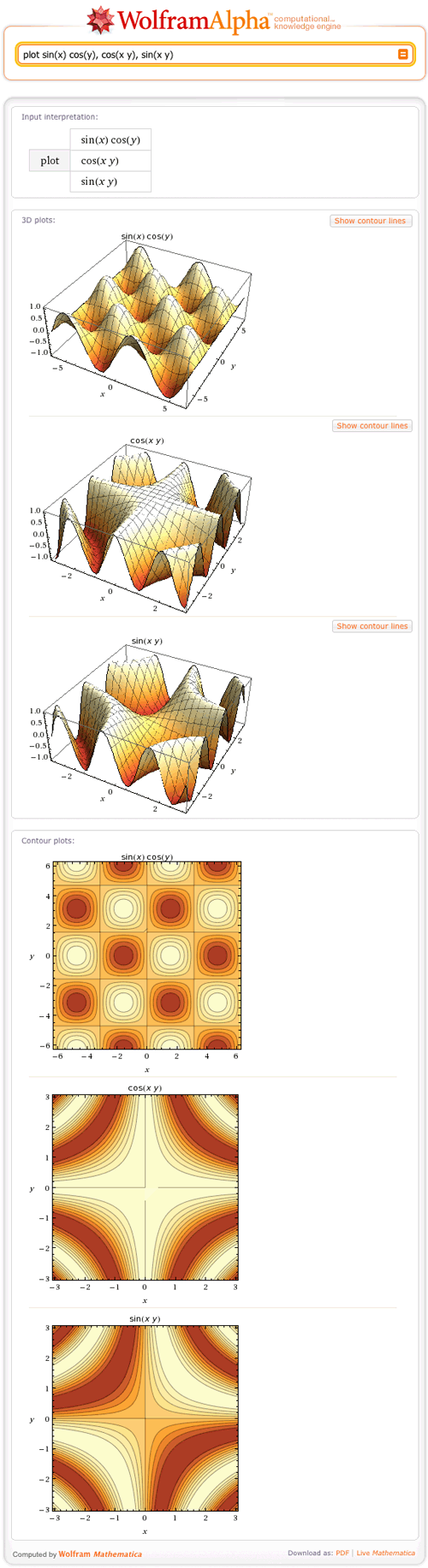Wolfram|Alpha will return an individual plot of each function in the list. Here are a couple more examples to test out:

A new feature in Wolfram|Alpha is the functionality to plot the real and imaginary parts of complex-valued bivariate functions. Here are a couple of examples:

In all of these examples Wolfram|Alpha returned a contour plot in addition to the 3D plot. A nice way to see the connection between the 3D and contour plots is to click the “Show contour lines” button. Note that the 3D and contour plots will always use the same plot range.

All of the 3D plots were made using Mathematica‘s function Plot3D; the contour plots were made using ContourPlot. In both cases the Mathematica code for generating the images can be found by clicking the plots.

You have now had the opportunity to view the plotting abilities of Wolfram|Alpha in 2D and 3D planes. Still not convinced? Try to plot your favorite function in Wolfram|Alpha, and be sure to share your results with us!

amazing…

Posted by thay May 17, 2011 at 3:06 pm

I think you should put all Mathematica on WolframAlpha 😀
It is amazing to have all those features in such a free website.
thanks wolfram.

Posted by Holmez May 17, 2011 at 3:47 pm

A boon to dress designers!

Posted by Brian Gilbert May 18, 2011 at 3:46 am

Sam, congrats … excellent job!! … The whole thing would be more than completed with some comments on plotting functions given implicitly. Cheers.

Posted by German Titiv May 25, 2011 at 12:42 am

Posted by mrego May 25, 2011 at 10:18 am

See what an amazing plot I have got
http://www.wolframalpha.com/input/?i=plot+x^sin%28y^2%29%3D+ln%28x*e^sinx%29

Posted by Harsh Gupta May 27, 2011 at 2:36 pm

Can you explain your inequality plots?

For example I do not understand http://www.wolframalpha.com/input/?i=plot+%28x%2By%29%3C5

Posted by Colleen Young May 29, 2011 at 6:09 am

The shaded area evaluates to true for that function – looks pretty standard to me. Did you mean “how does it choose what range to display when you haven’t given it context” ? I guess the same magic that it uses for the other graphs.

Posted by Ethan June 7, 2011 at 6:36 pm

Hi, I was wondering if it is possible to plot complex graphs in Wolfram Alpha…ie arg(z-3i)-arg(z+4)=?/2 ? If so, how? If not, it’d be nice if you added the feature! 😀

Posted by RUGRLN June 17, 2011 at 12:01 pm

Thanks for the reply. Am I missing something here?

http://www.wolframalpha.com/input/?i=plot+%28x%2By%29%3C5 looks like x+y< 7.5 to me.

I would expect x+y < 5 to look like this:

http://bit.ly/iDMuAL

ie to go through (5,0) and (0,5)

Posted by Colleen Young July 5, 2011 at 11:44 am

I wonder if it is possible to plot parametric surface here? I tried but did not succeed.

I wanted a sphere with this input:

parameric plot3D (cos(theta)*sin(phi), sin(theta)*sin(phi),cos(phi))

But no sphere is produced.

Posted by haowire September 5, 2011 at 9:49 pm

Can you find specific points on a graph? like at X=0.25 Y=?

Posted by Volkan Ceylan September 8, 2011 at 3:09 am

Hi!
How can I ask alpha to keep the range of y e.g y=0..1, however divide this interval to the finer grids. I mean instead of dividing y to 0, 0.02, 0.04, 0.06, 0.08, 1; divide it to 0, 0.002, 0.004,…,1

Posted by Maryam November 19, 2011 at 7:57 am

Hi!
Is there a way to force alpha plot the full vertical range? Similar to PlotRange->Full in real Mathematica?

Thanks!!

Posted by \yaroslav January 15, 2012 at 8:42 am

plot ? sin(x cos(y))^2

Posted by Danuke March 1, 2012 at 9:03 pm

In the 3D plot is it possible to plot the real function only i.e. for the domain, which guarantees that f(x, y) is real. The “real part” of the plot (2x-y)^(1/4) is for the whole R^2, I’d like it to be for 2x > y.

Posted by Eve April 12, 2012 at 11:37 am

How to change ratio of height and width of a plot in wolframalpha? AspectRatio function doesnt work.

Posted by Scyler September 11, 2012 at 2:18 pm

I was wondering how you got the axes labels to show up? Whenever I attempt to plot on Wolfram|Alpha they do not show up..

Posted by Stephanie February 20, 2014 at 7:01 pm

Is there a way to plot a two variable function and a 3 variable function on a single graph (ex: a line and a plane)?

Posted by josh March 13, 2014 at 10:04 pm

how can i plot some points on the XOY plane using the computer? secondly, how can i draw a pie chart using the computer?

Posted by stansfield July 4, 2014 at 2:03 pm

Why isn’t it possible for Wolfram Alpha to plot points or planes in 3D space?

Posted by Spencer September 14, 2014 at 8:39 pm

Hello, thank you for comment. Actually, it is possible for Wolfram|Alpha to perform both of those functions. Please see these two examples:

Posted by The W|A Team September 18, 2014 at 11:31 am

actually i got the output for 3 variables( v[i,j] , x[i] , y[i,j] ) ,how can i draw surface plot using this output.

Posted by varunkumar m September 15, 2014 at 11:32 pm

actually i got the output for 3 variables( v[i,j] , x[i] , y[i,j] ) ,how can i draw surface plot using this output.
and here i dont have expression for v[i,j] in terms of x[i] and y[i,j].

Posted by varunkumar m September 15, 2014 at 11:34 pm

how to plot a time varying function in 3D????
ex f(x,y,t)

Posted by Ankit November 7, 2014 at 3:52 am